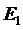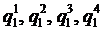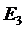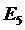16-reel slot machines

2. Case B – different numbers of stops and symbol distributions on the reels

2.1  Event– A specific symbol four times on a payline

The probability ofis, whereare the basic probabilities of that symbol occurring on the reels respectively.

2.3  Event– A specific symbol exactly three times on a payline

The probability ofis,whereare the basic probabilities of that symbol occurring on the reels respectively.

2.5  Event– Any combination of two specific symbols on a payline

The probability ofis, whereare the basic probabilities of the two symbols occurring on the reels respectively.